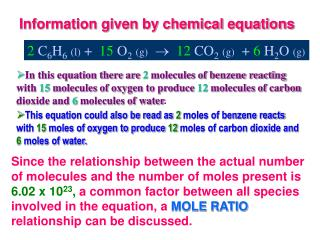Download PresentationInformation given by chemical equations

# Information given by chemical equations - PowerPoint PPT PresentationDownload Presentation## Information given by chemical equations

- - - - - - - - - - - - - - - - - - - - - - - - - - - E N D - - - - - - - - - - - - - - - - - - - - - - - - - - -
##### Presentation Transcript

1. Information given by chemical equations 2 C6H6(l) +15O2(g)12CO2(g) +6H2O (g) • In this equation there are 2 molecules of benzene reacting with 15 molecules of oxygen to produce 12 molecules of carbon dioxide and 6 molecules of water. • This equation could also be read as 2 moles of benzene reacts with 15 moles of oxygen to produce 12 moles of carbon dioxide and 6 moles of water. Since the relationship between the actual number of molecules and the number of moles present is 6.02 x 1023, a common factor between all species involved in the equation, a MOLE RATIO relationship can be discussed.

2. Information given by chemical equations 2 C6H6(l) +15O2(g)12CO2(g) +6H2O (g) The MOLE RATIO for benzene and oxygen is 2 : 15. It can be written as: 2 moles C6H6or as 15 moles of O2 15 moles O2 2 moles of C6H6 The MOLE RATIO for oxygen and carbon dioxide is 15 : 12. It can be written as: 12 moles CO2or as 15 moles of O2 15 moles O2 12 moles of CO2 • NOTE:The MOLERATIO is used for converting moles of one substance into moles of another substance. Without the balanced equation there is no other relationship between two different compounds.

3. Using the mole ratio to relate the moles of one compound to the moles of another compound is the part of chemistry called STOICHIOMETRY !!!!! 2 H2(g)+ O2(g)2 H2O (g) Q. How many mole of hydrogen are necessary to react with 2 moles of oxygen in order to produce exactly 4 moles of water? A. 2 mol O2 (2 moles H2 / 1 mole O2) = 4 mole H2

4. STOICHIOMETRY The Stoichiometry Flow Chart Use mole ratio from equation Use Molar mass (A) Use Molar mass (B)

5. STOICHIOMETRY 2 H2(g) + O2(g) 2 H2O (g) Q1. How many moles of hydrogen are necessary to react with 15.0 g of oxygen? A. 15.0g O2(1 mole O2)(2 mole H2)= 0.938 moles H2 32.0 g 1 mole O2 Q2. How many grams of hydrogen are necessary to react with 15.0 g of oxygen? A. 15.0g O2 (1 mole O2) ( 2 mole H2) ( 2.016 g H2) = 1.89 g H2 32.0 g 1 mole O21 mole H2

6. STOICHIOMETRY 2 H2(g) + O2(g) 2 H2O (g) Q3. How many grams of water are produced from 15.0 g of oxygen? A.15.0g O2 (1 mole O2) ( 2 mole H2O) ( 18.0 g H2O) =16.9 g H2O 32.0 g 1 mole O21 mole H2O Q4. How much hydrogen and oxygen is needed to produce 25.0 grams of water? A. 25.0g H2O (1 mole H2O)(2 mole H2)(2.016 g H2)= 2.80 g H2 18.0 g 2 mole H2O1 mole H2 A. 25.0g H2O (1 mole H2O)(1 mole O2)(32.0 g O2)= 22.2 g O2 18.0 g 2 mole H2O1 mole O2 Notice that the Law of Conservation of Mass still applies.

7. How many grams of solid are formed when 10.0 g of lead reacts with excess phosphoric acid? • Write the chemical equation: Pb + H3PO4 ? You recognize that this is a single displacement (replacement) reaction. So Pb (a metal) will displace (replace) H (the cation). Pb + H3PO4 Pb3(PO4)2 + H2 2. Balance the equation: 3Pb+2 H3PO4 Pb3(PO4)2 + 3 H2 3. Make a list under the appropriate substance 3Pb+2 H3PO4 Pb3(PO4)2 (s) + 3 H2 (g) 10.0gm=? Start with what is given: 10.0gPb (1 mole Pb)(1 mole Pb3(PO4)2)(811 g Pb3(PO4)2) = 13.1 g Pb3(PO4)2 207 g Pb 3 mole Pb1 mole Pb3(PO4)2

8. PRACTICE PROBLEM # 18 14.20g 1. How many grams of gas can be produced from 0.8876 moles of HgO? 2 HgO  2 Hg + O2 2. How many moles of fluorine are required to produce 12.0 grams of KrF6? Given the equation: Kr + 3 F2 KrF6 3. How many grams of Na2CO3 will be produced from the thermal decomposition of 250.0 g of NaHCO3? 4. How many grams of CO2 can be produced by the reaction of 75.0 grams of C2H2 with excess oxygen? 5. Cu + 2 AgNO3 Cu(NO3)2 + 2 Ag. How many grams of silver is produced when 125.0 g of copper is reacted with excess silver nitrate solution? 0.182 mol 157.7 g 254 g 424.9 g

9. GROUP STUDY PROBLEM # 18 ______1. How many grams of liquid product can be produced from 3.55 moles of HgO? 2 HgO 2 Hg + O2 ______2. How many moles of fluorine are required to produce 3.0 grams of KrF6? Given the equation: Kr + 3 F2 KrF6 ______3. How many grams of Na2CO3 will be produced from the decomposition of 20.0g of NaHCO3? ______4. How many grams of O2 are needed to combust 55.0 grams of C2H4? ______5. Cu + 2 AgNO3 Cu(NO3)2 + 2 Ag. How many grams of silver is produced when 50.0 g of copper is reacted with excess silver nitrate solution?